# Lecture 3: Gravitational Potential and N-Body Equations I.

## Conservative Force Fields

In a one-dimensional system it is always possible to define a potential energy corresponding to any given f(x); let

(1)whereis an arbitrary position at which. Different choices ofproduce potential energies differing by an additive constant; this constant has no influence on the dynamics of the system.

In a space ofdimensions the analogous path integral,

(2)may depend on the exact route taken from pointto; if it does, a unique potential energy cannot be defined. One condition for this integral to be path-independent is that the integral of the force f(x) around all closed paths vanishes. An equivalent condition is that there is some function U(x) such that

(3)Force fields obeying these conditions are conservative. The gravitational field of a stationary point mass is the simplest example of a conservative field; the energy released in moving from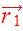to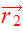with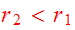is exactly equal to that consumed in moving back fromto.

## Gravitational Potential

In simulation projects it's natural to work with the path integral of the acceleration rather than the force; this integral is the potential energy per unit mass or gravitational potential,, and the potential energy of a test mass m is just. For an arbitrary mass density, the potential is

(4)whereis the gravitational constant and the integral is taken over all space. Poisson's equation provides another way to express the relationship between density and potential:

(5)Note that this relationship is linear; ifgeneratesandgeneratesthen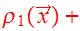generates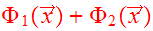. Gauss's theorem relates the mass within some volume V to the gradient of the field on its surface:

(6)where the infinitesimal vectoris an element of surface area with an outward-pointing normal vector.

## Spherical Potentials

Consider a spherical shell of mass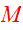:

(a) the acceleration inside the shell vanishes

(b) and the acceleration outside the shell is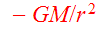.

From these results, it follows that the potential of an arbitrary spherical mass distribution is

(7)where the enclosed mass is

(8)### Elementary examples

A point of mass:

(9)This is known as a Keplerian potential since orbits in this potential obey Kepler's three laws. If G=1 is set, the velocity of a circular orbit at radius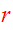isA uniform sphere of massand radius:

(10a)(10b)where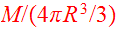is the mass density. Outside the sphere the potential is Keplerian, while inside it has the form of a parabola; both the potential and its derivative are continuous at the surface of the sphere.

## Galactic Potential, Scales and Units

Galactic potential is the collective self-consisten field of all stars within the galaxy. It is determined by the distribution functionwhich accounts for the mechanical state of the galaxy.

Sun is located in our Milky Way which hasvisible stars and aboutsolar masses of gas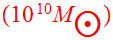with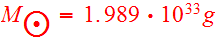. In comparison,. Gas has little effect on main features of galactic dynamics.

Most of the stars in the galaxy travel on nearly circular orbits in a thin disk whose radius is of the order ofand thickness of the order ofwith. Typical circular speed of stars is of the order of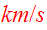and the time required to complete a galactic orbit atis about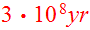. The dispersion in velocities is about. Age of the galaxy. Typical disk star (like our Sun) has completed over 30 revolutions. Galaxy is in steady state. Why and how is explained by the steady state solution of the distribution function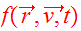from the collisionless Boltzmann equation.

### Scales and units

Consider first a practical choice on units for galaxy collisions:The gravitational constant is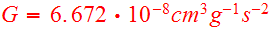in cgs units. Typical galaxy size is measured in tens of kiloparsecs, large scale structure of the universe is measured in Megaparsecs. Let us determine the value of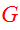in the new galactic units:

(11)This value of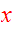is an inconvenient value forto carry in simulations. Let us try galactic units which set. One possible illustration isIn these units

(12)In these units we can usein the simulations. With this choice two units are arbitrary, the third unit is fixed. There is great flexibility to interpret simulation results in physical units at the very end of the runs with dimensionless numbers.astronomy units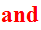Kepler orbits.Kepler orbits (1 earth year)one Saturn year